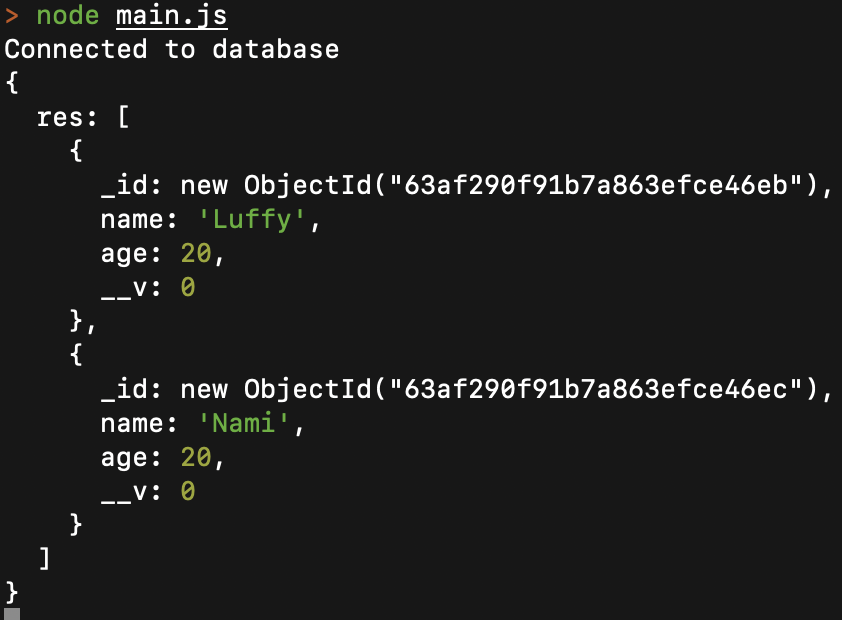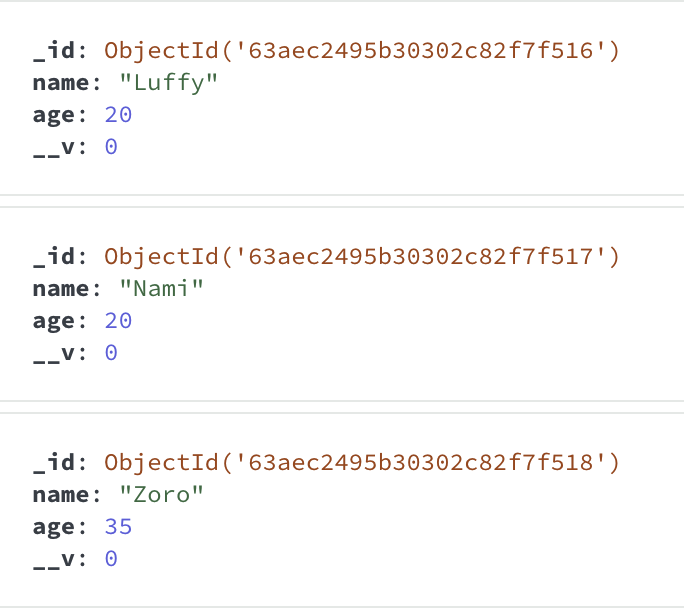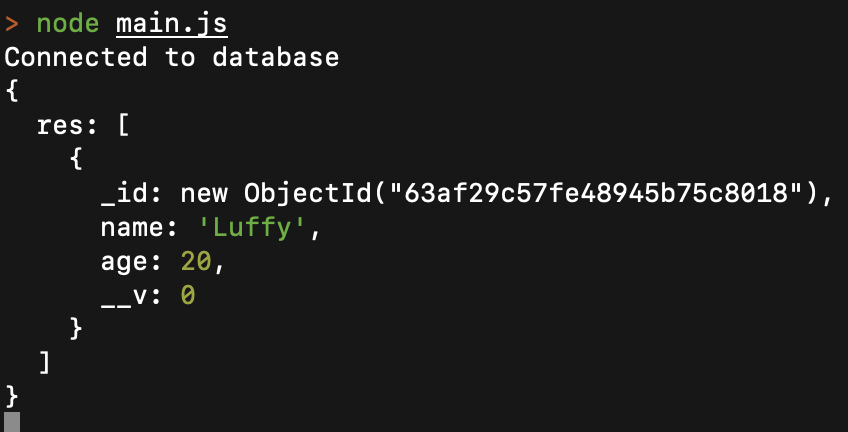Open in App
Not now

# Mongoose Query.prototype.equals() API

• Last Updated : 09 Jan, 2023

The Mongoose Query API equals() method is used to find the documents with fields’ values equal to the value passed in its parameter “val”, and return the response as an array of documents.

Syntax:

`Query.prototype.equals(val)`

Parameters: It accepted the following parameters as mentioned above and described below:

• val: A string that identifies the field’s value to match the existing documents.

Return type: It returns a Query object as a response.

Creating node application And Installing Mongoose:

Step 1: Create a node application using the following command:

```mkdir folder_name
cd folder_name
npm init -y
touch main.js```

Step 2: After completing the Node.js application, Install the required module using the following command:

`npm install mongoose`

Example 1: In this example, we will use this method to find documents whose “age” have a value equal to “20”.

Filename: main.js

## Javascript

 `const mongoose = require(``'mongoose'``) ` ` `  `// Database connection ` `mongoose.connect(``'mongodb://localhost:27017/query-helpers'``, ` `    ``{ ` `        ``dbName: ``'event_db'``, ` `        ``useNewUrlParser: ``true``, ` `        ``useUnifiedTopology: ``true` `    ``}, err => err ? console.log(err) ` `        ``: console.log(``'Connected to database'``)); ` ` `  `const personSchema = ``new` `mongoose.Schema({ ` `    ``name: { ` `        ``type: String, ` `    ``}, ` `    ``age: { ` `        ``type: Number, ` `    ``} ` `}); ` ` `  `const personsArray = [ ` `    ``{ ` `        ``name: ``'Luffy'``, ` `        ``age: 20 ` `    ``}, ` `    ``{ ` `        ``name: ``'Nami'``, ` `        ``age: 20, ` `    ``}, ` `    ``{ ` `        ``name: ``'Zoro'``, ` `        ``age: 35 ` `    ``} ` `] ` ` `  `const Person = mongoose.model(``'Person'``, personSchema); ` ` `  `(async () => { ` `    ``await Person.insertMany(personsArray); ` `    ``const res = await Person.where(``'age'``).equals(20) ` `    ``console.log({ res }); ` `})()`

Step to Run Application: Run the application using the following command from the root directory of the project:

`node main.js`

Output:GUI Representation of the  Database using MongoDB Compass:Example 2: In this example, we will use this method to find documents whose “name” have a value equal to “Luffy”.

Filename: main.js

## Javascript

 `const mongoose = require(``'mongoose'``) ` ` `  `// Database connection ` `mongoose.connect(``'mongodb://localhost:27017/query-helpers'``, ` `    ``{ ` `        ``dbName: ``'event_db'``, ` `        ``useNewUrlParser: ``true``, ` `        ``useUnifiedTopology: ``true` `    ``}, err => err ? console.log(err) ` `        ``: console.log(``'Connected to database'``)); ` ` `  `const personSchema = ``new` `mongoose.Schema({ ` `    ``name: { ` `        ``type: String, ` `    ``}, ` `    ``age: { ` `        ``type: Number, ` `    ``} ` `}); ` ` `  `const personsArray = [ ` `    ``{ ` `        ``name: ``'Luffy'``, ` `        ``age: 20 ` `    ``}, ` `    ``{ ` `        ``name: ``'Nami'``, ` `        ``age: 20, ` `    ``}, ` `    ``{ ` `        ``name: ``'Zoro'``, ` `        ``age: 35 ` `    ``} ` `] ` ` `  `const Person = mongoose.model(``'Person'``, personSchema); ` ` `  `(async () => { ` `    ``await Person.insertMany(personsArray); ` `    ``const res = await Person.where(``'name'``).equals(``'Luffy'``) ` `    ``console.log({ res }); ` `})()`

Step to Run Application: Run the application using the following command from the root directory of the project:

`node main.js`

Output:GUI Representation of the  Database using MongoDB Compass:My Personal Notes arrow_drop_up
Related Articles